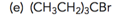Problem: Draw a bond-line structure for each of the following compounds:

FREE Expert Solution
81% (298 ratings)
Problem Details

Draw a bond-line structure for each of the following compounds:What scientific concept do you need to know in order to solve this problem?

Our tutors have indicated that to solve this problem you will need to apply the Condensed Structural Formula concept. You can view video lessons to learn Condensed Structural Formula Or if you need more Condensed Structural Formula practice, you can also practice Condensed Structural Formula practice problems .

What is the difficulty of this problem?

Our tutors rated the difficulty of Draw a bond-line structure for each of the following compoun... as medium difficulty.

What professor is this problem relevant for?

Based on our data, we think this problem is relevant for Professor Ternansky's class at UCSD.

What textbook is this problem found in?

Our data indicates that this problem or a close variation was asked in . You can also practice practice problems .